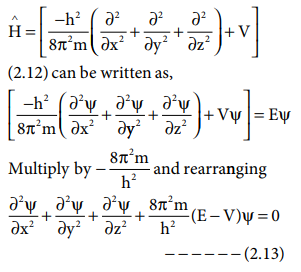Home | | Chemistry 11th std | Quantum mechanical model of atom - Schrodinger Equation

# Quantum mechanical model of atom - Schrodinger Equation

In classical mechanics the physical state of the particle is defined by its position and momentum.

Quantum mechanical model of atom ŌĆō Schrodinger Equation:

The motion of objects that we come across in our daily life can be well described using classical mechanics which is based on the NewtonŌĆÖs laws of motion. In classical mechanics the physical state of the particle is defined by its position and momentum. If we know both these properties, we can predict the future state of the system based on the force acting on it using classical mechanics. However, according to HeisenbergŌĆÖs uncertainty principle both these properties cannot be measured simultaneously with absolute accuracy for a microscopic particle such as an electron. The classical mechanics does not consider the dual nature of the matter which is significant for microscopic particles. As a consequence, it fails to explain the motion of microscopic particles. Based on the Heisenberg's principle and the dual nature of the microscopic particles, a new mechanics called quantum mechanics was developed.

Erwin Schr├Čdinger expressed the wave nature of electron in terms of a differential equation. This equation determines the change of wave function in space depending on the field of force in which the electron moves. The time independent Schr├Čdinger equation can be expressed as,Where H Ōł¦ is called Hamiltonian operator,

╬© is the wave function and is a funciton of position co-ordinates of the particle and is denoted as ╬©(x, y,z) E is the energy of the systemThe above schr├¢dinger wave equation does not contain time as a variable and is referred to as time independent Schr├Čdinger wave equation. This equation can be solved only for certain values of E, the total energy. i.e. the energy of the system is quantised. The permitted total energy values are called eigen values and corresponding wave functions represent the atomic orbitals.

## Main features of the quantum mechanical model of atom

1. The energy of electrons in atoms is quantised

2. The existence of quantized electronic energy levels is a direct result of the wave like properties of electrons. The solutions of Schr├Čdinger wave equation gives the allowed energy levels (orbits).

3. According to Heisenberg uncertainty principle, the exact position and momentum of an electron can not be determined with absolute accuracy. As a consequence, quantum mechanics introduced the concept of orbital. Orbital is a three dimensional space in which the probability of finding the electron is maximum.

4. The solution of Schr├¢dinger wave equation for the allowed energies of an atom gives the wave function Žł, which represents an atomic orbital. The wave nature of electron present in an orbital can be well defined by the wave function Žł.

5. The wave function Žł itself has no physical meaning. However, the probability of finding the electron in a small volume dxdydz around a point (x,y,z) is x proportional to |Žł(x,y,z)|2 dxdydz |Žł(x,y,z)|2 is known as probability density and is always positive.

Tags : Equation, Main features , 11th Chemistry : UNIT 2 : Quantum Mechanical Model of Atom
Study Material, Lecturing Notes, Assignment, Reference, Wiki description explanation, brief detail
11th Chemistry : UNIT 2 : Quantum Mechanical Model of Atom : Quantum mechanical model of atom - Schrodinger Equation | Equation, Main features